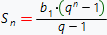# Series sum online calculator

In order to calculate the series sum, one need simply make summation over all the elements of the series. For instance:In the example above the summation procedure was very simple, as far as it was done the finity number of times. But what should we do if the upper summation bound is infinity? For instance, we need to find the sum of the following series:As in the previous example, we can write this sum like this:But what should we do next?! At this stage it is necessary to introduce the partial series sum conception. So, the partial series sum (denotes Sn) is called the sum of the first n terms of the series. I.e. in our case:Knowing that, we can calculate the sum of the initial series as the limit of the partial series sum:Therefore, to calculate series sum, one need somehow to find the expression of the partial series sum (Sn). In our case the series is the decreasing geometric series with ratio 1/3. It is known that the sum of the first n elements of geometric series can be calculated by the formula:where b1 - is the first element of the geometric series (in our case it equals to 1) and q is the geometric series ratio (in our case 1/3). Therefore, the partial sum Sn for our series equals to:Then the sum of our series (S) accoding the definition given above equals to:The examples above is very simple. Usually to calculate the series sum, one need to make much more effort, and the main difficulty is to find the partial series sum. The online calculator below was created on the basis of the Wolfram Alpha, and it is able to find sum of highly complicated series. In addition, when the calculator fails to find series sum is the strong indication that this series is divergent (the calculator prints the message like "sum diverges"), so our calculator also indirectly helps to get information about series convergence.

To find you series sum, you need to choose the series variable, lower and upper bounds and also input the expression for n-th term of the series.

## Choose expression input type:

Expression input type:

## Choose integration variable and summation limits:

Summation variable

Lower summation bound: .

Upper summation bound: .